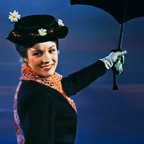# Expialidocious# Expialidocious Lyrics

"George, I think we should listen"
"Wha- ? I- oh, very well"

Never give, and then I'll never be dissing songs
Us, sing this
Never give, and then I'll never be dissing songs
Us, sing this
Never give, and then I'll never be dissing songs
Us, sing this
Never give, and then I'll never be dissing songs
Us, sing this

Six-oh-two
See the light, it's the light
Six-oh-two
See the light, it's the -

Never give, and then I'll never be dissing songs
Us, sing this
Never give, and then I'll never be dissing songs
Us, sing this
Never give, and then I'll never be dissing songs
Us, sing this
Never give, and then I'll never be dissing songs
Us, sing this

Search of deep satisfaction!

Six-oh-two
Six-oh-two
Six-oh-two
Six-oh-two
It's the light
Six-oh-two
It's the light
Six-oh-two
It's the light
Six-oh-two
It's the light
Six-oh-two
It's the light
Six-oh-two
It's the light
Six-oh-two
It's the light
Six-oh-two
It's the light
Six-oh-two
It's the light
Six-oh-two
It's the light
Six-oh-two
It's the light
Six-oh-two
It's the light
Six-oh-two

Never give, and then I'll never be dissing songs
Us, sing this
Never give, and then I'll never be dissing songs
Us, sing this

Us, and then never give us this box and then never give us and then never give us this box
Us, and then never give us this box and then never give us and then never give us this box
Us, and then never give us this box and then never give us and then never give us this box
Us, and then never give us this box and then never give us and then never give us this box

Search of deep satisfaction!